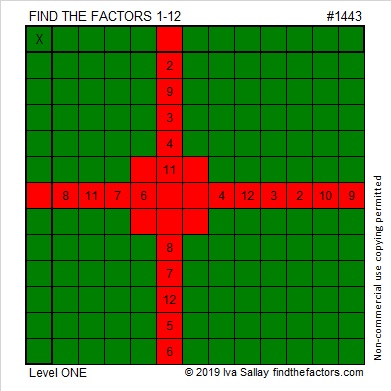# 1443 A Simple Gift for You

This level one puzzle is my simple gift to you. Yes, you can solve it, and you don’t even have to wait until December 25th to discover all the factors and products to be found inside!That was puzzle number 1443. Here are some facts about that number.

• 1443 is a composite number.
• Prime factorization: 1443 = 3 × 13 × 37
• 1443 has no exponents greater than 1 in its prime factorization, so √1443 cannot be simplified.
• The exponents in the prime factorization are 1, 1, and 1. Adding one to each exponent and multiplying we get (1 + 1)(1 + 1)(1 + 1) = 2 × 2 × 2 = 8. Therefore 1443 has exactly 8 factors.
• The factors of 1443 are outlined with their factor pair partners in the graphic below.(38-1)(38+1) = 1443 so 1443 is one number away from 38² = 1444.

Actually, 1443 is the difference of two squares in four different ways:
38² – 1² = 1443
62²- 49² = 1443
242² – 239² = 1443
722² – 721² = 1443

1443 is the hypotenuse of FOUR Pythagorean triples:
93-1440-1443 which is 3 times (31-480-481)
468-1365-1443 which is (12-35-37) times 39
555-1332-1443 which is (5-12-13) times 111
957-1080-1443 which is 3 times(319-360-481)

This site uses Akismet to reduce spam. Learn how your comment data is processed.